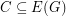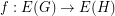Petersen coloring conjecture

 Importance: High ✭✭✭
 Author(s): Jaeger, Francois
 Subject: Graph Theory » Coloring » » Edge coloring
 Keywords: cubic edge-coloring Petersen graph
 Prize: none
 Posted by: mdevos on: March 7th, 2007
Conjecture   Letbe a cubic graph with no bridge. Then there is a coloring of the edges ofusing the edges of the Petersen graph so that any three mutually adjacent edges ofmap to three mutually adjancent edges in the Petersen graph.

This extrordainary conjecture asserts that in a very strong sense, every bridgeless cubic graph has all of the cycle-space properties posessed by the Petersen graph. If true, this conjecture would imply both The Berge-Fulkerson conjecture and The five cycle double cover conjecture.

Ifis a graph andwe say thatis a binary cycle if every vertex in the graphhas even degree. Ifis a graph andis a map, we say thatis cycle-continuous if the pre-image of every binary cycle is a binary cycle. The following conjecture is an equivalent reformulation of the Petersen coloring conjecture.

Conjecture  (Petersen coloring conjecture (2))   Every bridgeless graph has a cycle-continuous mapping to the Petersen graph.

Question

For which bridgeless cubic graphs has this been checked for?

Re:

It is trivially true for all that are 3-edge-colorable -- which is the vast majority. Among the rest, I checked it using computer and lists of snarks for all graphs upto 34 vertices. (And some more -- e.g. all flower-snarks.)

Best wishes, Robert